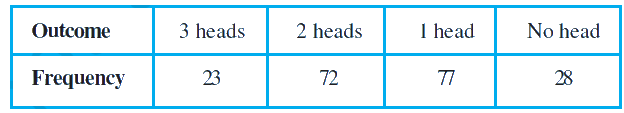# Ex.15.1 Q4 Probability Solution - NCERT Maths Class 9

## Question

Three coins are tossed simultaneously $$200$$ times with the following frequencies of different outcomesIf the three coins are simultaneously tossed again, compute the probability of $$2$$ heads coming up.

Video Solution
Probability
Ex exercise-15-1 | Question 4

## Text Solution

What is known?

Total number of tosses and frequencies of different outcomes.

What is unknown?

Probability of $$2$$ heads coming up when three coins are tossed simultaneously.

Reasoning:

The empirical probability $$P(E)$$ of an event $$E$$ happening, is given by:

\begin{align}{P}({E})=\frac{ \begin{bmatrix} \text { Number of trials in which }\\ \text{the event happened } \end{bmatrix} }{ \begin{bmatrix} \text { The total number} \\ \text{of trials } \end{bmatrix} }\end{align}

Use probability to derive the solution where

Probability of a particular outcome

\begin{align} & { = \frac{\begin{bmatrix}{\text{No of time of a particular }} \\ {\text{outcome occurs }}\end{bmatrix} }{{{\text{Total number of tosses}}}}} \end{align}

Steps:

Given total number of tosses $$= 200$$

No of $$2$$ heads outcomes $$= 72$$

Probability [of head outcomes]

\begin{align} & { = \frac{ {\text{Number of times }} {\text{heads occur}} }{{{\text{Total number of tosses}}}}} \\ &=\frac{72}{200} \\ &=\frac{9}{25} \end{align}

Learn from the best math teachers and top your exams

• Live one on one classroom and doubt clearing
• Practice worksheets in and after class for conceptual clarity
• Personalized curriculum to keep up with school